Function Repository Resource:

# TukeyMedianPolish

Find row and column effects in a data matrix by repeatedly subtracting the median

Contributed by: Sjoerd Smit
 ResourceFunction["TukeyMedianPolish"][mat] repeatedly removes row and column medians from matrix mat until it no longer changes. ResourceFunction["TukeyMedianPolish"][mat,"prop"] specifies what property should be returned. ResourceFunction["TukeyMedianPolish"][] represents an operator form of ResourceFunction["TukeyMedianPolish"] that can be applied to an expression.

## Details and Options

Matrix mat has to be numerical.
Median polishing is a robust exploratory data analysis method to find row and column effects in a matrix of data similar to two-way ANOVA. The main idea is that the elements of the matrix are of the form mi,j=c+xi+yj+ϵi,j. In this model, the xi are called the row effects, yj the column effects, c the overall effect and the ϵi,j are the residuals. In order to estimate these effects, it is assumed that all of these quantities (except the constant c) average out to 0 over i and/or j. However, since the amount of data is limited, the Mean is not a good location estimator to use and instead the Median is used as a more robust alternative. Other location estimators can be specified if necessary.
To estimate c, xi and yj, the algorithm first subtracts the medians from the columns of mat and adds them to the yj and also subtracts the median from the xi vector and adds it to c. Then the algorithm repeats the process for the rows (adding the medians to the xi) and the yj (adding its median to c again). This process is iterated until convergence is reached.
The argument "prop" can either be "Matrix" (default) or "PropertyAssociation".
ResourceFunction["TukeyMedianPolish"] takes the following options:
 "LocationEstimator" Median location estimator to use MaxIterations 100 maximum number of iterations to use Tolerance Scaled[10-4] maximum (scaled) difference between two successive steps before the algorithm terminates "ConvergenceTest" Automatic function that tests if convergence has been reached Compiled False whether to use function compilation to speed up the computation
If a custom "LocationEstimator" is specified, it should behave in the same way as Median and Mean when applied to a matrix, meaning that locEst[matrix] should produce the same result as locEst/@Transpose[matrix].
If an option value other than Automatic is specified for "ConvergenceTest", the Tolerance option will be ignored. The algorithm will evaluate sametest[medians,current] after each iteration, with medians being the median values computed in this step and current being the current output result if the algorithm stops. When the function returns True, the algorithm stops.
For "ConvergenceTest" Automatic, the Tolerance option determines the stopping criterion. If Tolerance Scaled[tol] is specified, the default test is Function[Max@Abs[#1] < tol*Max@Abs[#2]]. If the tolerance is instead specified as Tolerance tol, the default test is Function[Max@Abs[#1]<tol].
If the Compiled option is used, extra compilation options can be propagated into Compile by specifying Compiled{True,opts…}.

## Examples

### Basic Examples (7)

Define a matrix:

 In:=Out=Calculate the median polish:

 In:=Out=The medians of the columns of the polished residuals are (approximately) zero:

 In:=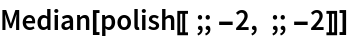Out=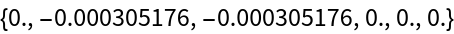As are the medians of the rows:

 In:=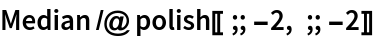Out=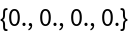The median of the column effects is (approximately) zero:

 In:=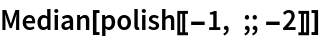Out=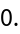As is the median of the rows effects:

 In:=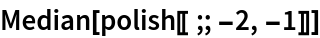Out=The overall effect is not zero, since the original matrix consists of numbers ≥ 0:

 In:=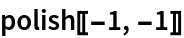Out=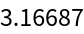### Scope (1)

The second argument can be used to request the result to be returned as an Association instead of a matrix:

 In:=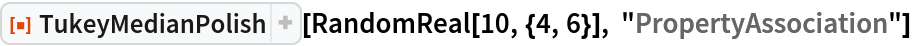Out=### Options (9)

#### LocationEstimator (1)

Compare the results when different location estimators are used:

 In:=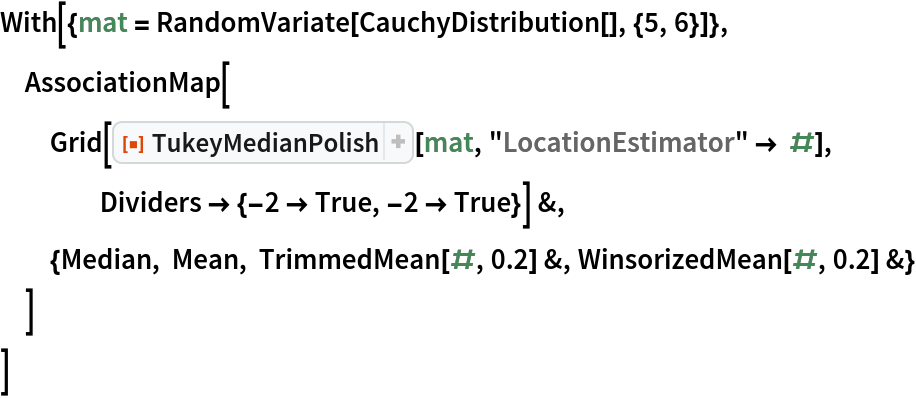Out=#### Tolerance (2)

The medians of the row and column residuals depends on the specified tolerance:

 In:=Out=Specify a smaller tolerance to bring the medians closer to zero:

 In:=Out=Specify an absolute tolerance:

 In:=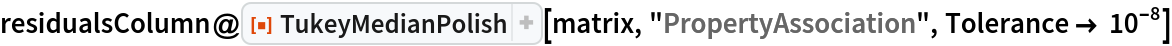Out=Use the operator form to map over lists of matrices:

 In:=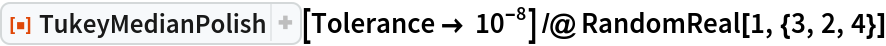Out=#### MaxIterations (2)

Tolerances may not be met if the matrix is large or the tolerance is small:

 In:=Out=Increasing the MaxIterations can solve this:

 In:=Out=#### Compiled (4)

The computation speed can be increased significantly with compilation:

 In:=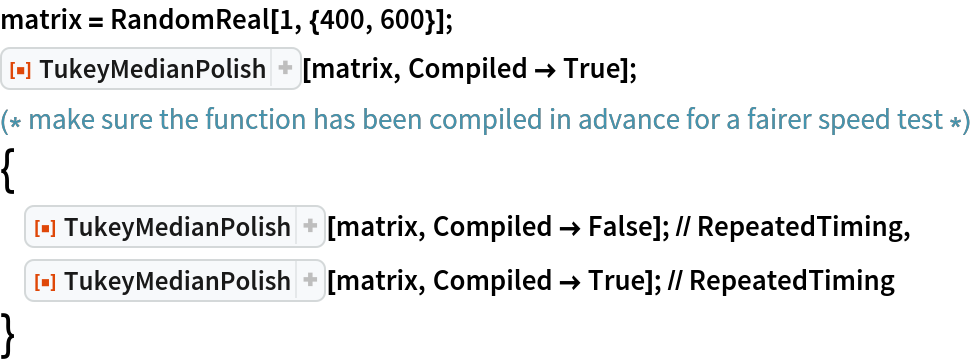Out=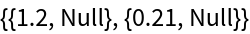Compiled computations will be done at machine precision, while the default computation will retain precision:

 In:=Out=The operator form returns a CompiledFunction if the option is used:

 In:=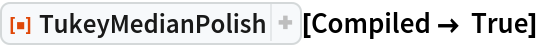Out=You can set the Listable attribute to the compiled function so that it automatically threads over multiple inputs:

 In:=Out=### Possible Issues (1)

Using exact numbers can produce very large expressions:

 In:=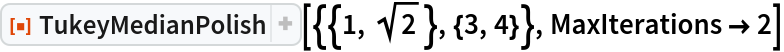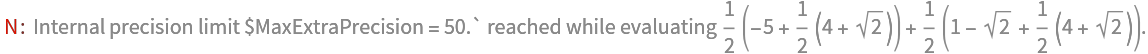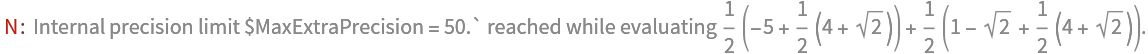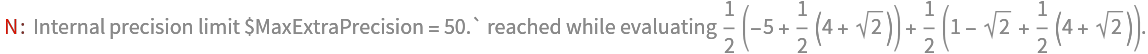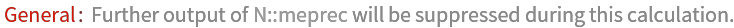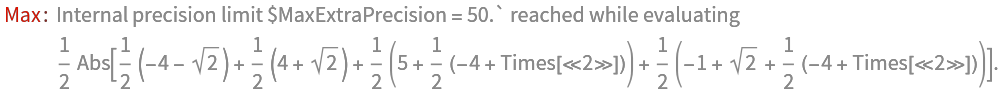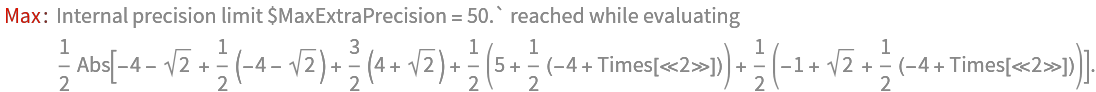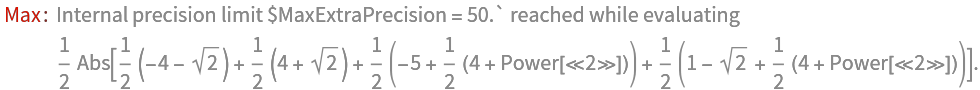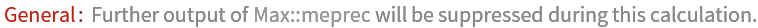Out=Sjoerd Smit

## Version History

• 1.0.0 – 10 March 2020